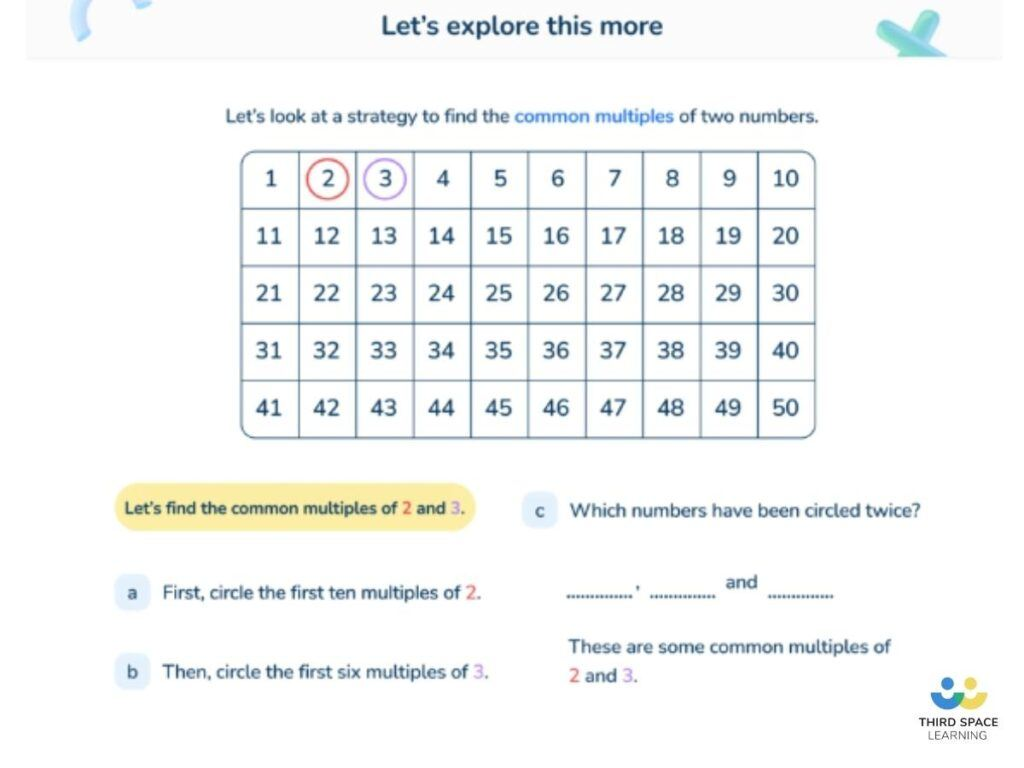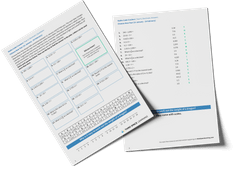Math teaching support you can trustone-on-one tutoring sessionsschools supported

[FREE] Fun Math Games and Activities Packs for Kindergarten to Grade 5

Ready to go, printable packs that teachers can use in the classroom.

# What Is A Multiple: Explained For Teachers, Parents and Kids

Make learning what is a multiple, simple for elementary children. Here is a step by step guide to support teaching multiples in elementary school. A secure knowledge of multiplication facts is essential in calculating multiples.

### What is a multiple?

A multiple of a number is the result when that number is multiplied by an integer. Examples of a multiple of 14 could be 14 (14 x 1), 28 (14 x 2) or 140 (14 x 10).

The definition of multiple can also be described as numbers in a given multiplication table – for example, 5, 10, 15 and 20 are all multiplications of 5; they are also all multiples of 5. Even numbers are all multiples of 2. There is a multiple of every number.

Children often confuse multiples with factors. Read more about factors of a number and the greatest common factor (also known as the highest common factor).

### When do children learn about multiples in the common core?

Children are expected to know the first 12 multiples of the numbers 1-12 by the end of 4th grade. This is gradually taught throughout elementary school.

Kindergarten:

• Count in multiples (also known as ‘skip counting’) of ones and tens, within 100.

• Count in multiples of 2’s, 10’s and 100’s, within 120.
• Add on and subtract in multiples of 10, within 100.

• Count in multiples (skip-count) by 5’s, 10’s and 100’s, within 1,000.

• Count in multiples of 6, 7, 9, 25 and 1000
• Multiply one-digit whole numbers by multiples of 10 (i.e. 9 x 50, 6 x 30)

• Find factor pairs for a whole numbers in the range 1-100
• Solve problems involving multiplication and division including using their knowledge of factors and multiples
• Use factors and multiples to recognize equivalent fractions and simplify where appropriate

Alongside the regular multiplication facts, multiples of 25, 50, 100 and 1000 are also taught, as these are useful when working with fractions and decimals, as well as units of measurement.

• Identify common factors, common multiples and prime numbers
• Use common multiples to express fractions in the same denomination
• Solve problems involving unequal sharing and grouping using knowledge of fractions and multiples

### What is the lowest common multiple?

The lowest common multiple (LCM), also referred to as the least common multiple, is the smallest multiple shared between two given numbers.

For example, the lowest common multiple of 9 and 12 is 36 as it is the smallest multiple that both given numbers share (9 x 4 = 36 and 12 x 3 = 36). Lowest common multiples can be used to express fractions in the same denomination, which is useful when they are being added or subtracted.A simple visual representation of how to identify common multiples in a Third Space Learning online tutoring lesson.

### How do multiples relate to other areas of math?

Multiples and factors are often taught together: while factors are the result when you divide a number by an integer (with no remainder), multiples are the result when you multiply a number by an integer.

In 4th grade geometry, children are taught multiples of 90 degrees (90 = quarter turn; 180 = half a turn, or a straight line; 270 = three-quarter turn; 360 = full turn, or a circle). As previously mentioned, finding common multiples is also useful when expressing fractions in the same denomination.

### Multiples of numbers in real life

Multiples are useful when organizing a number into groups – for example, 25 is a multiple of 5: 25 children could be organized into groups of 5; 25 cents could be paid in 5 nickels.

Common multiples are also useful in real life contexts – for example, if burgers come in packs of 8 but burger buns come in packs of 6, finding a common multiple (24) would help you work out how many of each pack to buy (3 and 4 respectively) without wasting any.

### 5 multiple practice questions and answers

• 1. What’s the lowest common multiple of 8 and 12?
• 2. Chen uses these digit cards: 5 6 9. She makes a two-digit number and a one-digit number. She multiplies them together. Her answer is a multiple of 10. What could Chen’s multiplication be?
• 3. Write all the common multiples of 3 and 8 that are less than 50.
• 4. In the circles, write a multiple that belongs to each set. One has been done for you.

• 5. Here is a diagram for sorting numbers. Write one number in each box. One is done for you.

1. 24
2. 96 x 5
3. 24, 48
4. a) 120, 140, 160 or 180
b) 210, 240 or 270
c) 320 or 360
5. Top right: 3, 6, 9, 12, 18 etc.
Bottom left: 5, 10, 20, 25, 35 etc.
Bottom right: 1, 2, 4, 7, 8 etc.

What are the multiples of 12?

Some of the multiples of 12 are 12, 24, 36, 48, 60, 72, 84, 96, 108, 120, 132, 144, but there are many many more. The multiples of 12 are achieved by multiplying any other number by 12.

What is a multiple and a factor?

A multiple is the product of two numbers. A factor is a number that fits exactly into a given number, or divides a particular number with no remainder. A factor is always a positive integer (whole number).

How do you explain multiples?

A multiple of any given number can be achieved by multiplying that number by any integer (whole number)

Looking for more advice on how to explain other mathematical concepts to your students? Take a look at our Math Dictionary for Kids

Do you have students who need extra support in math?
Give your fourth and fifth grade students more opportunities to consolidate learning and practice skills through personalized elementary math tutoring with their own dedicated online math tutor.

Each student receives differentiated instruction designed to close their individual learning gaps, and scaffolded learning ensures every student learns at the right pace. Lessons are aligned with your state’s standards and assessments, plus you’ll receive regular reports every step of the way.

The content in this article was originally written by primary school teacher Sophie Bartlett and has since been revised and adapted for US schools by elementary math teacher Christi Kulesza.##### Sophie Bartlett
Year 6 Teacher
Sophie teaches mixed age classes at a small school in central England. She is a self confessed grammar pedant and number nerd so we've welcomed her as a regular author and editor for Third Space Learning.
xx

#### [FREE] Fun Math Games and Activities Packs for Kindergarten to Grade 5

Individual packs for Kindergarten to Grade 5 containing fun math games and activities to complete independently or with a partner.

The activities are designed to be fun, flexible and suitable for a range of abilities.